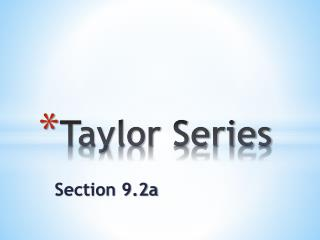DownloadDownload PresentationTaylor Series

# Taylor Series

Télécharger la présentation## Taylor Series

- - - - - - - - - - - - - - - - - - - - - - - - - - - E N D - - - - - - - - - - - - - - - - - - - - - - - - - - -
##### Presentation Transcript

1. Taylor Series Section 9.2a

2. Do Now – Exploration 1 on p.469 Construct a polynomial with the following behavior at : Since , the constant coefficient is Since , the coefficient of is Since , the coefficient of is (The 2 in the denominator is needed to cancel the factor of 2 that results from differentiating )

3. Do Now – Exploration 1 on p.469 Construct a polynomial with the following behavior at : Since , the coefficient of is Since , the coefficient of is The polynomial:

4. Now we will use the technique from this exploration to construct polynomials that approximate functions by emulating their behavior at 0… Construct a polynomial that matches the behavior of at through its first four derivatives. That is, at at at at at

5. Construct a polynomial that matches the behavior of at through its first four derivatives. This is similar to the first exploration, except that first we need to find out what the numbers are:

6. Construct a polynomial that matches the behavior of at through its first four derivatives. This is similar to the first exploration, except that first we need to find out what the numbers are: The polynomial: Notice a pattern from our first two examples? The coefficient of the term in the polynomial we seek is divided by .

7. Construct a polynomial that matches the behavior of at through its first four derivatives. The polynomial: This is the fourth order Taylor polynomial for the function at (look familiar?... Section 9.1!!!). If we continued this pattern, we could construct an infinite geometric power series, which would be the Taylor series generated by the given function at the given point.

8. Definition: Taylor Series Generated by f at x = 0 (Maclaurin Series) Let f be a function with derivatives of all orders throughout some open interval containing 0. Then the Taylor series generated by f at x = 0 is This series is also called the Maclaurin series generated by f. The partial sum is the Taylor polynomial of order n for f at x = 0.

9. More Explorations Construct the seventh order Taylor polynomial and the Taylor series for at . First, evaluate and its first seven derivatives at : This pattern (0, 1, 0, –1…) will keep repeating forever…

10. More Explorations Construct the seventh order Taylor polynomial and the Taylor series for at . The unique seventh order Taylor polynomial that matches all these derivatives at : Look at and read about “Beauty Bare” on p.472… To form the Taylor series, just keep going:

11. More Explorations Construct the seventh order Taylor polynomial and the Taylor series for at . Taylor polynomial: Taylor series: Did anyone see a shortcut to this answer??? Differentiate the Taylor polynomial for sine term-by-term…

12. Definition: Taylor Series Generated by f at x = a Let f be a function with derivatives of all orders throughout some open interval containing a. Then the Taylor series generated by f at x = a is The partial sum is the Taylor polynomial of order n for f at x = a.

13. More Explorations Find the Taylor series generated by at . The Taylor series: For graphical support, graph the following two functions in [–1, 4] by [–10, 50]:

14. More Explorations On the intersection of their intervals of convergence, Taylor series can be added, subtracted, and multiplied by constants and powers of x, and the results are once again Taylor series. For example, the Taylor series for f(x) + g(x) is the sum of the Taylor series for f(x) and the Taylor series for g(x)… We obtain the Maclaurin series for (x)sin(x) by multiplying all the terms of the Maclaurin series for sin(x) by x… Etc… Lastly, note the Table of Maclaurin Series on p.477. You will use these series as building blocks for constructing other series…